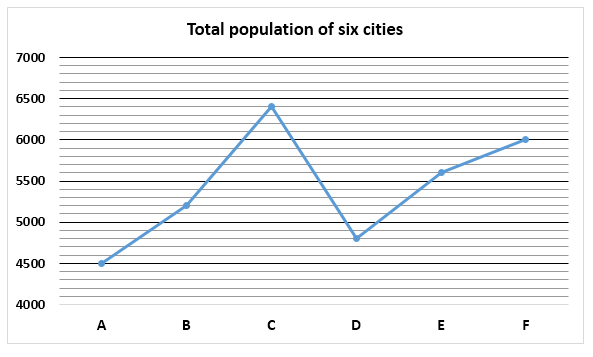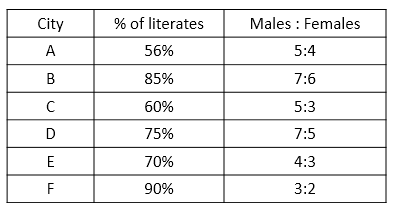# IBPS RRB PO Mains Quantitative Aptitude Questions 2019 (Day-02)

Dear Aspirants, Our IBPS Guide team is providing new series of Quantitative Aptitude Questions for IBPS RRB PO Mains 2019 so the aspirants can practice it on a daily basis. These questions are framed by our skilled experts after understanding your needs thoroughly. Aspirants can practice these new series questions daily to familiarize with the exact exam pattern and make your preparation effective.

Check here for IBPS RRB PO Mains Mock Test 2019

Check here for IBPS RRB Clerk Mock Test 2019

### Click Here to Subscribe Crack High Level Puzzles & Seating Arrangement Questions PDF 2019 Plan

[WpProQuiz 6811]

1) A, B and C started a business by investing 1500, 2500 and 3500 respectively. They invested for 4, 5 and 6 months respectively.  If 5.2% of the total profit goes to charity and A’s share is Rs. 7200, then the total profit is?

b) 40000

c) 50000

d) 28000

e) None of these

2) The product of the age of A, B and C is 5760. The ratio of ages of A and B is 3: 5 and A and C is 1: 2. Find sum of their age?

b) 58

c) 56

d) 54

e) None of these

3) The ratio of boys and girls in a school is 4: 5. If 20% of boys and 30% of girls like Tamil, then find the ratio of boys and girls who did not like Tamil?

a) 32: 35

b) 35: 32

c) 1: 1

d) 4: 3

e) None of these

4) The area of a square is 1764 sq cm whose side is twice the radius of a circle. The circumference of the circle is equal to breadth of a rectangle, if perimeter of rectangle is 564 cm. What is the area of rectangle?

a) 19800 cm2

b) 19600 cm2

c) 18900 cm2

d) 16900 cm2

e) None of these

5) The ratio of the two numbers is 7: 4, the LCM of the number is 224. Find the product of this number?

a) 1684

b) 1456

c) 1792

d) 1487

e) None of these

Directions (6 – 10): Study the following information carefully and answer the questions given below:6) If ratio of literate and illiterate males in city B is 4:3 respectively, find the percentage of literate females in city B.

a) 50.22%

b) 75.12%

c) 33.33%

d) 65.13%

e) None of these

7) Out of the illiterate population of city D, 30% are females. Find the percentage of literate males in city D.

a) 60%

b) 55%

c) 70%

d) 45%

e) None of these

8) Find the respective ratio of number of males in city A number of illiterates in city C.

a) 123: 128

b) 125: 128

c) 123: 125

d) 125: 127

e) None of these

9) Find the total number of literates in all the cities together.

a) 20400

b) 21600

c) 22500

d) 23700

e) None of these

10) Find the difference between total number of males and total number of females in all the cities.

a) 5300

b) 4200

c) 6200

d) 4800

e) None of these

Direction (1-5) :

Profit ratio = (1500 * 4): (2500 * 5): (3500 * 6)

= 12: 25: 42

Total profit = (7200/12) * (79/94.8) * 100 = 50000

A: B: C = 3: 5: 6 (3x, 5x, 6x)

3x * 5x * 6x = 90x3 = 5760

x = 4

Required sum = 3 * 4 + 5 * 4 + 6 * 4 = 56

Boys: Girls = 4: 5

Unlike Tamil = 4 * 80/100 : 5 * 70/100

= 32: 35

Side of the square = √1764 = 42

Radius of circle = 42/2 = 21

Circumference = 2 * 22/7 * 21 = 132

Length of the rectangle = 564/2 – 132 = 150

Area of the rectangle = 132 * 150 = 19800 cm2

HCF = 224/28 = 8

Product = 224 * 8 = 1792

Direction (6-10) :

Number of males in city B = 7/13 x 5200 = 2800

Number of females in city B = 6/13 x 5200 = 2400

Total number of literates in city B = 85/100 x 5200 = 4420

Literate males in city B = 4/7 x 2800 = 1600

Literate females in city B = 2400 – 1600 = 800

Percentage of literate females = 800/2400 x 100 = 33.33%

Illiterate population of city D = (100 – 75)/100 x 4800

= 25/100 x 4800

= 1200

Number of illiterate females = 30/100 x 1200 = 360

Number of illiterate males = 1200 – 360 = 840

Number of males in city D = 7/12 x 4800 = 2800

Number of literate males = 2800 – 840 = 1960

Percentage of literate males = 1960/2800 x 100

= 70%

Number of males in city A = 5/9 x 4500 = 2500

Number of illiterates in city C = (100 – 60)/100 x 6400

= 40/100 x 6400

= 2560

Required ratio = 2500: 2560 = 125: 128

Number of literates in city A = 56/100 x 4500 = 2520

Number of literates in city B = 85/100 x 5200 = 4420

Number of literates in city C = 60/100 x 6400 = 3840

Number of literates in city D = 75/100 x 4800 = 3600

Number of literates in city E = 70/100 x 5600 = 3920

Number of literates in city F = 90/100 x 6000 = 5400

Required sum = 2520 + 4420 + 3840 + 3600 + 3920 + 5400 = 23700

Total number of males in all the cities = 5/9 x 4500 + 7/13 x 5200 + 5/8 x 6400 + 7/12 x 4800 + 4/7 x 5600 + 3/5 x 6000

= 2500 + 2800 + 4000 + 2800 + 3200 + 3600

= 18900

Total number of females in all the cities = 4/9 x 4500 + 6/13 x 5200 + 3/8 x 6400 + 5/12 x 4800 + 3/7 x 5600 + 2/5 x 6000

= 2000 + 2400 + 2400 + 2000 + 2400 + 2400

= 13600

Required difference = 18900 – 13600 = 5300